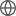Old Web
English
We report the discovery of an ultrahot Jupiter with an extremely short orbital period of $0.67247414\,\pm\,0.00000028$ days ($\sim$16 hr). The $1.347 \pm 0.047$ $R_{\rm Jup}$ planet, initially identified by the Transiting Exoplanet Survey Satellite (TESS) mission, orbits TOI-2109 (TIC 392476080): a $T_{\rm eff} \sim 6500$ K F-type star with a mass of $1.447 \pm 0.077$ $M_{\rm Sun}$, a radius of $1.698 \pm 0.060$ $R_{\rm Sun}$, and a rotational velocity of $v\sin i_* = 81.9 \pm 1.7$ km s$^{-1}$. The planetary nature of TOI-2109b was confirmed through radial velocity measurements, which yielded a planet mass of $5.02 \pm 0.75$ $M_{\rm Jup}$. Analysis of the Doppler shadow in spectroscopic transit observations indicates a well-aligned system, with a sky-projected obliquity of $\lambda = 1\overset{\circ}{.}7 \pm 1\overset{\circ}{.}7$. From the TESS full-orbit light curve, we measured a secondary eclipse depth of $731 \pm 46$ ppm, as well as phase-curve variations from the planet's longitudinal brightness modulation and ellipsoidal distortion of the host star. Combining the TESS-band occultation measurement with a $K_s$-band secondary eclipse depth ($2012 \pm 80$ ppm) derived from ground-based observations, we find that the dayside emission of TOI-2109b is consistent with a brightness temperature of $3631 \pm 69$ K, making it the second hottest exoplanet hitherto discovered. By virtue of its extreme irradiation and strong planet-star gravitational interaction, TOI-2109b is an exceptionally promising target for intensive follow-up studies using current and near-future telescope facilities to probe for orbital decay, detect tidally driven atmospheric escape, and assess the impacts of H$_2$ dissociation and recombination on the global heat transport.